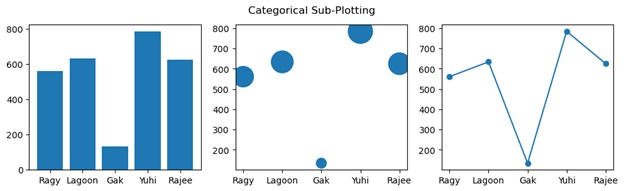# Python | Comparing Categorical Plotting Methods

Here, we are going to compare categorical plotting methods with the examples in Python.
Submitted by Anuj Singh, on July 24, 2020

In this tutorial, we are going to compare categorical plots with Bar, Scatter, and Line Plot through sub plotting. It will help us to decide which one is better for visualization and further use of similar applications.## Python code for comparing categorical plotting methods

```import matplotlib.pyplot as plt

names = ['Ragy', 'Lagoon', 'Gak', 'Yuhi', 'Rajee']
values = [561, 634, 134, 785, 625 ]

plt.figure(figsize=(12, 3))

plt.subplot(131)
plt.plot(names, values, '-o')
plt.suptitle('Categorical Sub-Plotting')
plt.subplot(132)
plt.scatter(names, values, s=values)
plt.subplot(133)
plt.bar(names, values)
plt.show()
```

Output:

```Output is as figure
```